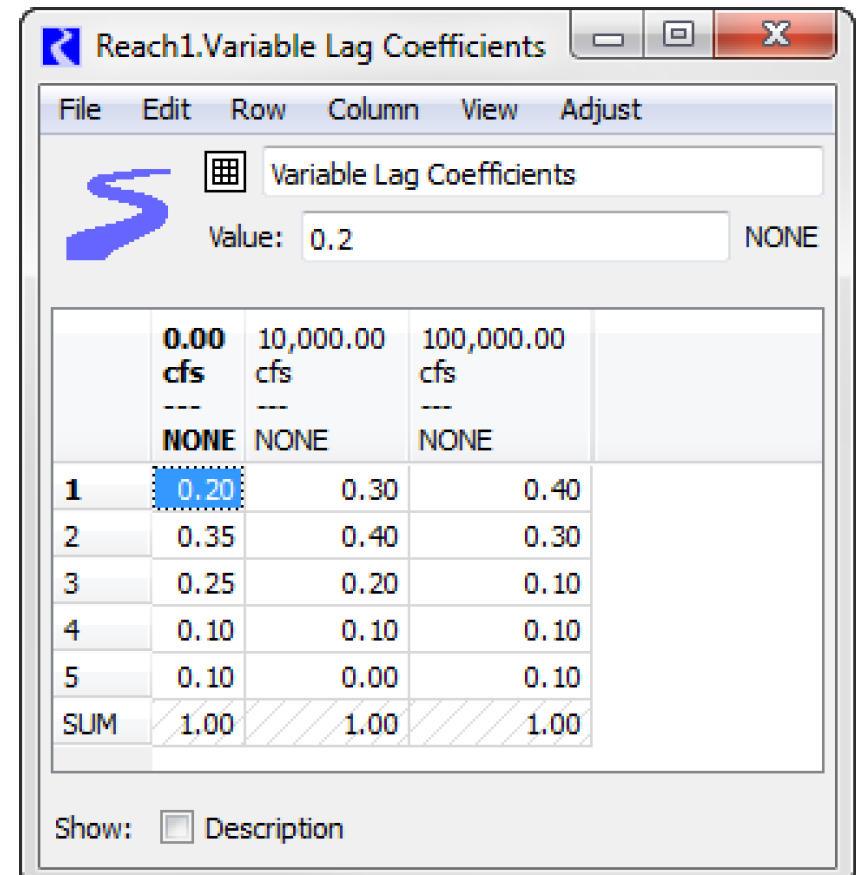Objects and Methods : Reach : User Methods : Alternative Routing on Subbasin
Alternative Routing on Subbasin
This method category is used to provide alternative routing coefficients for use in subbasin methods.None
This is the default method. No calculations are performed and there are no slots associated with this method.Variable Step Coefficients
This method is used to provide variable step coefficients for use in subbasin methods that aggregate routing coefficients for a control point. See Alternative Routing Coefficients Methods in USACE‑SWD Modeling Techniques for details on the variable routing. This method allows the reach to solve one way in simulation dispatching, but another way in these subbasin methods.
Slots Specific to This Method
Variable Lag Coefficients
Type: Table Slot
Units: Column Map Values - Flow, Table values - No Units
Description: A table defining the step response coefficients for each inflow threshold is shown.Information: This table has a column map, which means that each column has an associated numerical value (with units). This numerical value is displayed as the column label.
Columns are added and deleted from this table using the Column menu with the following options: Set Number of Columns, Append Column, Delete Column and Delete Last Column.
User units, scale, type, and precision for the column map (that is, the column heading values) are defined in the unit scheme for Flow unit types. Column map values are set from the Column, Set Column Value menu option.
When a column value is changed, the columns reorder to ensure the column values are increasing, from left to right.
The sum of coefficients in each column should equal 1.0. This can be verified by adding a summary row at the bottom of the table by selecting View, then Show Column Sum Row.
When used in the Variable Step Response method, the column map values are used as a stair-step lookup. For example, an Inflow of 500cfs uses the first column; an inflow of 10,000cfs uses the second column; and an inflow of 120,000cfs uses the third column. Therefore, the column map values need not bound the highest expected flows; flows greater than the largest column map value use the right-most column.
Note: The left-most set of coefficients should represent the minimum flow in the reach.
I/O: Required input
Revised: 01/11/2023